Ex 1.5

Chapter 1 Class 11 Sets (Term 1)
Serial order wise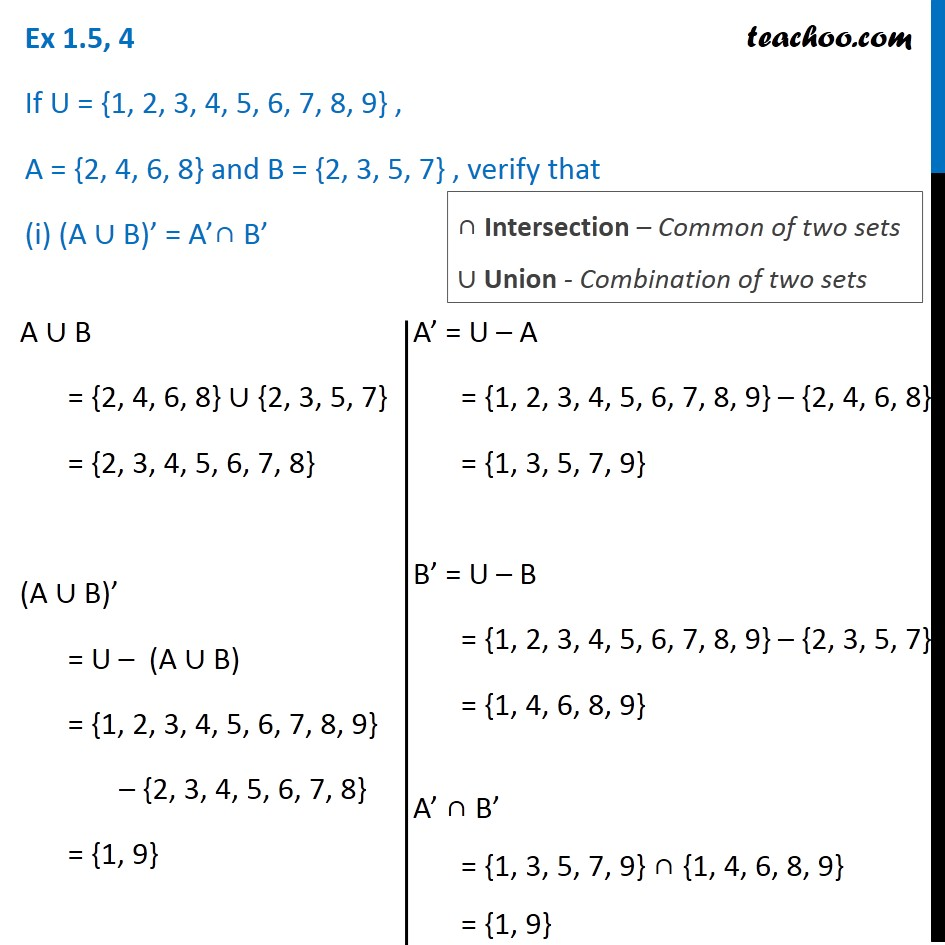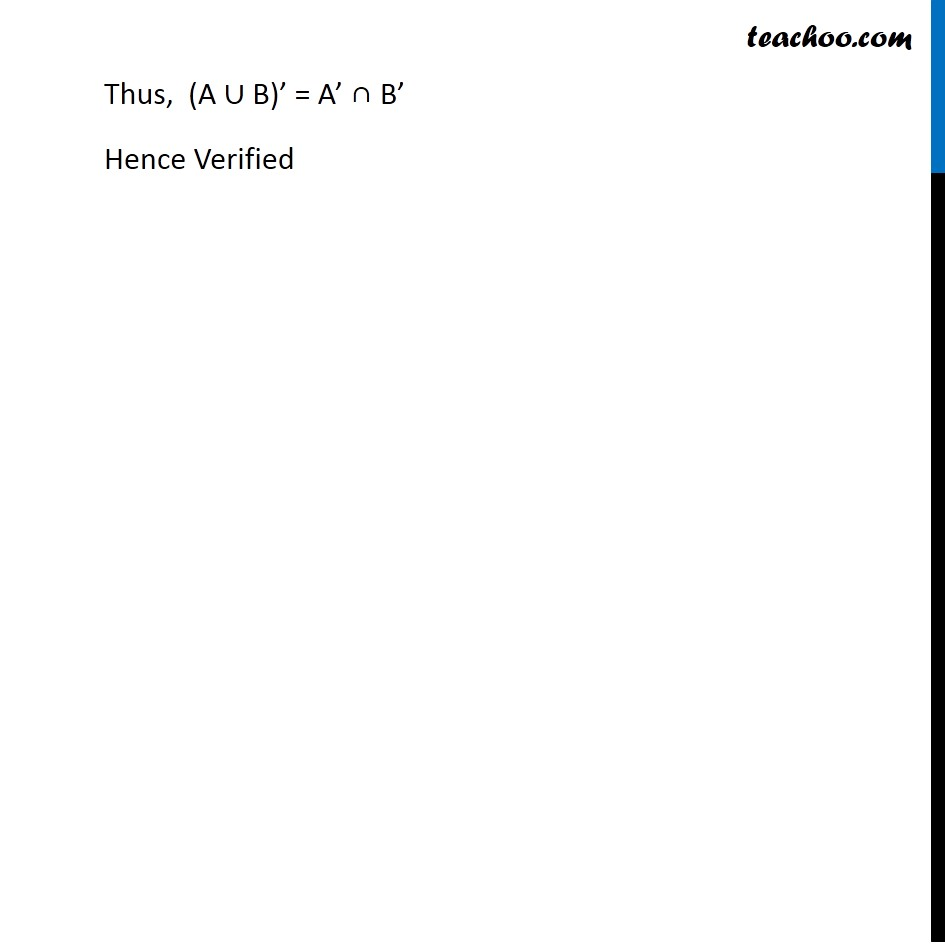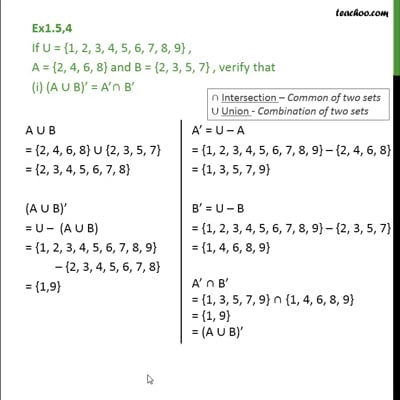This video is only available for Teachoo black users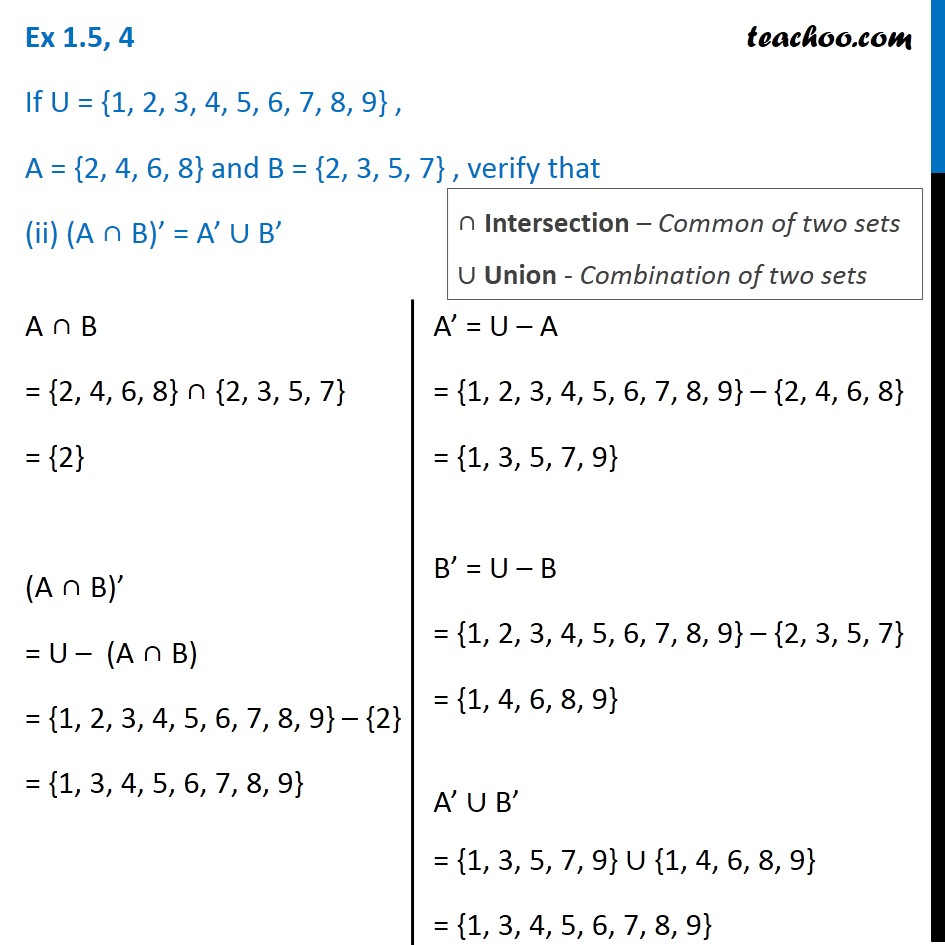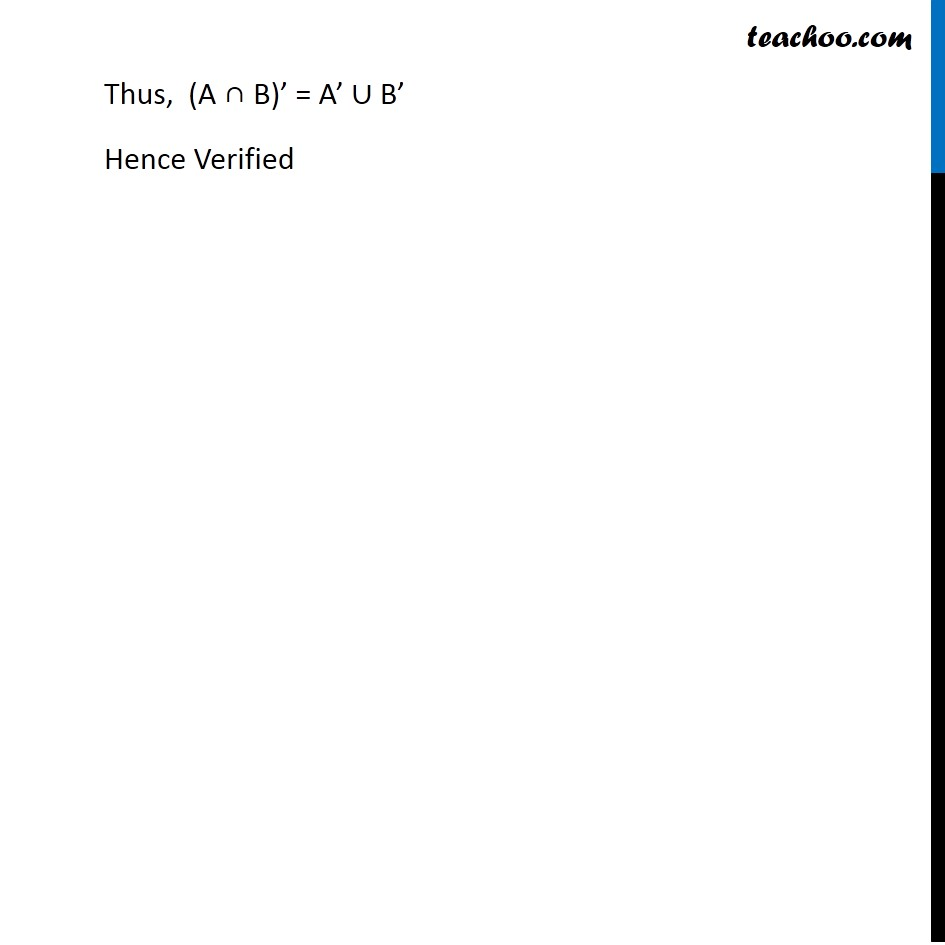### Transcript

Ex 1.5, 4 If U = {1, 2, 3, 4, 5, 6, 7, 8, 9} , A = {2, 4, 6, 8} and B = {2, 3, 5, 7} , verify that (i) (A ∪ B)’ = A’∩ B’ A ∪ B = {2, 4, 6, 8} ∪ {2, 3, 5, 7} = {2, 3, 4, 5, 6, 7, 8} (A ∪ B)’ = U – (A ∪ B) = {1, 2, 3, 4, 5, 6, 7, 8, 9} – {2, 3, 4, 5, 6, 7, 8} = {1, 9} A’ = U – A = {1, 2, 3, 4, 5, 6, 7, 8, 9} – {2, 4, 6, 8} = {1, 3, 5, 7, 9} B’ = U – B = {1, 2, 3, 4, 5, 6, 7, 8, 9} – {2, 3, 5, 7} = {1, 4, 6, 8, 9} A’ ∩ B’ = {1, 3, 5, 7, 9} ∩ {1, 4, 6, 8, 9} = {1, 9} ∩ Intersection – Common of two sets ∪ Union - Combination of two sets Thus, (A ∪ B)’ = A’ ∩ B’ Hence Verified Ex 1.5, 4 If U = {1, 2, 3, 4, 5, 6, 7, 8, 9} , A = {2, 4, 6, 8} and B = {2, 3, 5, 7} , verify that (ii) (A ∩ B)’ = A’ ∪ B’ A ∩ B = {2, 4, 6, 8} ∩ {2, 3, 5, 7} = {2} (A ∩ B)’ = U – (A ∩ B) = {1, 2, 3, 4, 5, 6, 7, 8, 9} – {2} = {1, 3, 4, 5, 6, 7, 8, 9} ∩ Intersection – Common of two sets ∪ Union - Combination of two sets A’ = U – A = {1, 2, 3, 4, 5, 6, 7, 8, 9} – {2, 4, 6, 8} = {1, 3, 5, 7, 9} B’ = U – B = {1, 2, 3, 4, 5, 6, 7, 8, 9} – {2, 3, 5, 7} = {1, 4, 6, 8, 9} A’ ∪ B’ = {1, 3, 5, 7, 9} ∪ {1, 4, 6, 8, 9} = {1, 3, 4, 5, 6, 7, 8, 9} Thus, (A ∩ B)’ = A’ ∪ B’ Hence Veraified